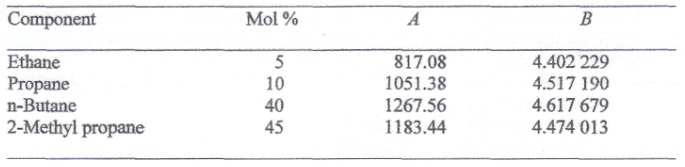Whether HCl is a base or an acid

Whether HCl is a base or an acid? Briefly state your comments?

E

Expert

##### Verified

HCl or hydrochloric acid, as the name entails, is an acid. However, it is considered a strong acid as it dissociates totally in water to form the H3O+ and Cl-.

Though, it can as well act as a base in reactions with acids stronger than it can similar to HClO4.

#### Related Questions in Chemistry

• ##### Q :Problem on bubble point The following

The following mixture of hydrocarbons is obtained as one stream in a petroleum refinery.##### Q :Question associated to vapour pressure

Choose the right answer from following. The vapour pressure lowering caused by the addition of 100 g of sucrose(molecular mass = 342) to 1000 g of water if the vapour pressure of pure water at 25degree C is 23.8 mm Hg: (a)1.25 mm Hg (b) 0.125 mm Hg (c) 1.15 mm H

• ##### Q :Donnan Membrane Equilibria The electric

The electric charge acquired by macromolecules affects the equilibrium set up across a semipermeable membrane.

Laboratory studies of macromolecule solutions as in osmotic pressure and dialysis studies confine the macromolecules to one compartment while allo

• ##### Q :Dipole moment of chlorooctane

Illustrate the dipole moment of chlorooctane?

• ##### Q :Vapour pressure of water Give me answer

Give me answer of this question. 5cm3 of acetone is added to 100cm3 of water, the vapour pressure of water over the solution: (a) It will be equal to the vapour pressure of pure water (b) It will be less than the vapour pressure of pure water

• ##### Q :What is Elevation in boiling point? The

The boiling of a liquid may be defused by the temperature at which its vapour pressure which is equal to atmospheric pressure. The effect of addition in a non-volatile solute on the boiling point shown and its solution containing non-volatile solute with tempe

• ##### Q :Molarity Give me answer of this

Give me answer of this question. If 20ml of 0.4N, NaoH solution completely neutralises 40ml of a dibasic acid. The molarity of the acid solution is:(a) 0.1M (b) 0.2M (c)0.3M (d)0.4M

• ##### Q :Number of mlecules in methane Can

Can someone please help me in getting through this problem. The total number of molecules in 16 gm of methane will be: (i) 3.1 x 1023 (ii) 6.02 x 1023 (iii) 16/6.02 x 1023 (iv) 16/3.0 x 1023

• ##### Q :Explain Solid Compound Formation. In

In some two component, solid liquid systems, a solid compound forms.

In systems in which the components have an interaction for such other, a solid state compound of the two compounds of the two components can form.

Formic acid and formaide form a solid state, one-to-one com

• ##### Q :Mole fraction of hydrogen Give me

Give me answer of this question. In a mixture of 1 gm H2 and 8 gm O2 , the mole fraction of hydrogen is: (a) 0.667 (b) 0.5 (c) 0.33 (d) None of these